## Sunday, October 21, 2012

### 9V\$SQL , V\$SQLTEXT,V\$SQLAREA, V\$SQL_PLAN

 V\$SQL All sql in the shared pool, does not include “group by “ , only has first 1000 char V\$SQLTEXT All sql in the shared pool,only has first 1000 char V\$SQLAREA All sql in the shared pool, keep complete sql but break into multiple rows V\$SQL_PLAN All the query plan in the library cache V\$SQL_WORKAREA show information about work areas used by SQL cursors. V\$SQL_WORKAREA_ACTIVE show the work areas currently allocated by the system.

#### Show top most often used SQL

`SELECT`
`  sql_text,`
`  executions`
`FROM`
`  (`
`    SELECT`
`      sql_text,`
`      executions,`
`      rank() over ( order by executions DESC) exec_rank`
`    FROM`
`      v\$sql`
`  )`
`WHERE`
`  exec_rank >10`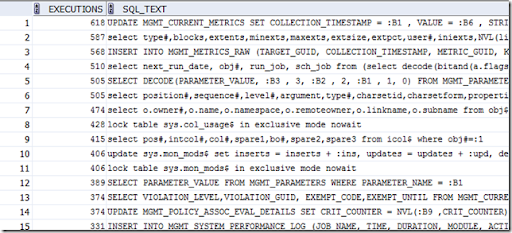#### show top IO intensive SQL ( physical IO)

`SELECT`
`  disk_reads,`
`  sql_text`
`FROM`
`  (`
`    SELECT`
`      sql_text,`
`      disk_reads,`
`      dense_rank() over ( order by disk_reads  DESC) disk_reads_rank`
`    FROM`
`      v\$sql`
`  )`
`WHERE`
`  disk_reads <=10;`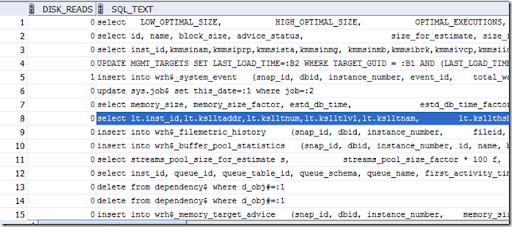`select b.username "USER NAME", a.disk_reads "DISK READ",a.executions EXECUTIONS,`
`a.disk_reads/decode( a.executions,0,1,a.executions) "READ_EXEC%",`
`a.sql_text "SQL TEXT"`
`from v\$sqlarea a, dba_users b`
`where a.parsing_user_id = b.user_id`
`order by a.disk_reads desc;`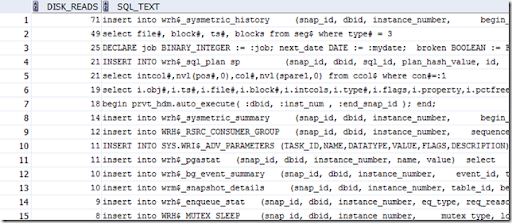#### show top logical IO SQL

`SELECT`
`  buffer_gets,`
`  sql_text`
`FROM`
`  (`
`    SELECT`
`      sql_text,`
`      buffer_gets,`
`      dense_rank() over ( order by buffer_gets  DESC) buffer_gets_rank`
`    FROM`
`      v\$sql`
`  )`
`WHERE`
`  buffer_gets <=10;`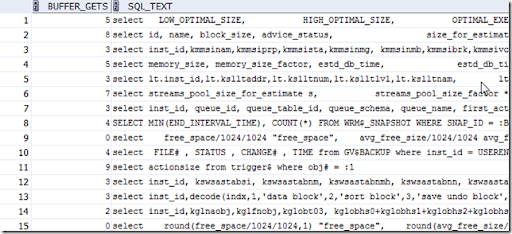#### Find the SQL for the expensive query

Here the expensive means either physical io or logical io large than 10000, the number could be differ depends on the environment.

Get the hash code first

`select hash_value,executions, buffer_gets,disk_reads, parse_calls`
`from v\$SQLAREA`
`where buffer_gets > 10000 or disk_reads > 10000`
`order by buffer_gets + 100 * disk_reads desc`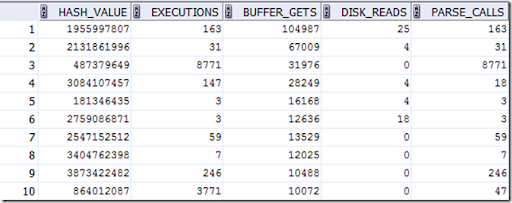Get the SQL

`select address,hash_value,piece,sql_text`
`from V\$sqltext`
`where hash_value = '487379649'`
`order by piece;`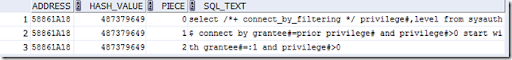Find the query plan

`select operation,options, object_name, cost `
`from v\$sql_plan`
`where address = '58861A18' and hash_value='487379649'`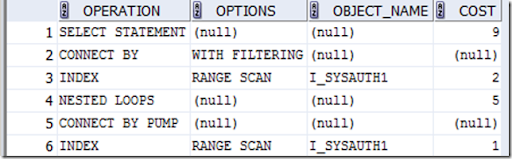#### Find the query plan for the current active user session

Find the active session

`select username,terminal,program,sql_hash_value,sql_address,type`
`from v\$session`
`where type not like 'BACKGROUND'`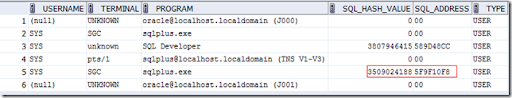`select operation,options, object_name, cost `
`from v\$sql_plan`
`where address = '5F9F10F8' and hash_value='3509024188'`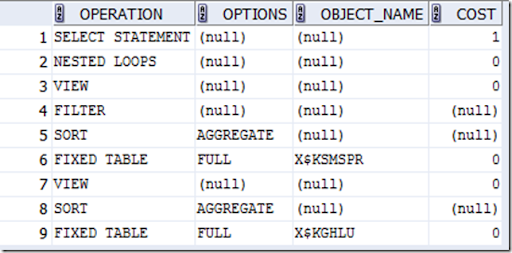Reference

http://www.kingstone.com.tw/book/book_page.asp?kmcode=2013120140585

1.Great post, Thank you!

2.nice and concise

3.4.5.6.SELECT
sql_text
FROM
(
SELECT
sql_text,
FROM
v\$sql
)
WHERE

should be : where disk_reads_RANK <=10

7.8.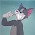9.